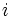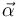# Parallel coordinate descent

## Definition

Parallel coordinate descent is a variant of gradient descent where we use a different learning rate in each coordinate. Explicitly, whereas with ordinary gradient descent, we define each iterate by subtracting a scalar multiple of the gradient vector from the previous iterate:

Ordinary gradient descent:$\vec{x}^{(k + 1)} = \vec{x}^{(k)} - \alpha^{(k)} \nabla f\left(\vec{x}^{(k)}\right)$

In parallel coordinate descent, we use a vector learning rate, i.e., we use a learning rate that could be different in each coordinate:

Parallel coordinate descent: for each coordinate$i$:$\vec{x}^{(k + 1)}_i = \vec{x}^{(k)}_i - \alpha^{(k)}_i \nabla f\left(\vec{x}^{(k)} \right)$

Alternatively, using coordinate-wise vector multiplication, we can describe the above as:$\vec{x}^{(k + 1)} = \vec{x}^{(k)} - \vec{\alpha}^{(k)} * \nabla f\left(\vec{x}^{(k)}\right)$

## Intuition behind choice of learning rate

For ordinary gradient descent, the learning rate$\alpha$ is typically chosen to be the reciprocal of the second derivative, or a suitable global bound on the second derivative. We may therefore expect that for parallel coordinate descent, we should choose, in each direction, the reciprocal of the second-order partial derivative of$f$ in that direction. In other words, we might expect that the vector$\vec{\alpha}$ is the vector with coordinates:$\alpha_i = \frac{1}{\frac{\partial^2 f}{\partial x_i^2}}$

The problem with this naive implementation is that if the coordinates are correlated, then we can overstep. To avoid overstepping, we can take the above expression and divide by an upper bound on the amount of correlation between coordinates. Fill this in later

## Types of parallel coordinate descent

Name Verbal description Is the rule stationary or dependent on number of iterations? Mathematical formalism Further comments
Parallel coordinate descent with constant learning rate (default meaning of parallel coordinate descent) Here, the learning rate vector is constant and independent of the number of iterations. Note that it could still differ between the different coordinates -- that's the point of parallel coordinate descent. Stationary$\vec{x}^{(k + 1)} = \vec{x}^{(k)} - \vec{\alpha} * \nabla f\left(\vec{x}^{(k)}\right)$,$\vec{\alpha}$ is the learning rate vector
Parallel coordinate descent with exact line search Here, we perform an exact line search in each coordinate; however, we still need to perform an overstep correction. Stationary
Parallel coordinate descent using Newton's method Here, we use Newton's method for optimization of a function of one variable; however, we still need to perform an overstep correction. Stationary